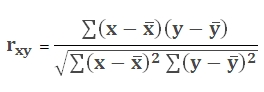# SQL Server RSQ function

Updated: 9 August 2010

Use RSQ_q to return the Pearson product moment correlation coefficient through data points in known-y's and known-x's. The r-squared value can be interpreted as the proportion of the variance in y attributable to the variance in x. The equation for the Pearson product moment correlation coefficient is:RSQ returns r2 which is the square of this correlation coefficient
Syntax
SELECT [wctStatistics].[wct].[RSQ_q] (
<@Known_y_Known_x_RangeQuery, nvarchar(4000),>)
Arguments
@Known_y_Known_x_RangeQuery
the select statement, as text, used to determine the known x- and y-values to be used in the RSQ_q calculation.
Return Types
float
Remarks
·         If the known-y dataset is empty or the known-x dataset is empty, then RSQ_q returns an error.
·         If the known-y dataset contains a different number of data points than the known-x dataset, then RSQ_q returns an error.
·         If the variance of dataset 1 or the variance of dataset 2 is zero, RSQ_q will return a divide by zero error.
·         For simpler queries and queries on de-normalized tables, consider using RSQ.
Examples

Calculate the RSQ value on a normalized table:
from #s1 a, #s1 b
where a.subject = ' + Char(39) + 'Math' + Char(39) + ' and
b.subject = ' + Char(39) + 'Science' + Char(39) + 'and
a.student = b.student')

This produces the following result

----------------------
0.999998988550504

(1 row(s) affected)

### SupportCopyright 2008-2023 Westclintech LLC         Privacy Policy        Terms of Service